# Helioprojective#

class sunpy.coordinates.Helioprojective(*args, **kwargs)[source]#

A coordinate or frame in the Helioprojective Cartesian (HPC) system, which is observer-based.

• The origin is the location of the observer.

• Tx (aka “theta_x”) is the angle relative to the plane containing the Sun-observer line and the Sun’s rotation axis, with positive values in the direction of the Sun’s west limb.

• Ty (aka “theta_y”) is the angle relative to the Sun’s equatorial plane, with positive values in the direction of the Sun’s north pole.

• distance is the Sun-observer distance.

This system is frequently used in a projective form without distance specified. For observations looking very close to the center of the Sun, where the small-angle approximation is appropriate, Tx and Ty can be approximated as Cartesian components.

A new instance can be created using the following signatures (note that if supplied, obstime and observer must be keyword arguments):

Helioprojective(Tx, Ty, obstime=obstime, observer=observer)
Helioprojective(Tx, Ty, distance, obstime=obstime, observer=observer)

Parameters:

Examples

>>> from astropy.coordinates import SkyCoord
>>> import sunpy.coordinates
>>> import astropy.units as u
>>> sc = SkyCoord(0*u.deg, 0*u.deg, 5*u.km,
...               obstime="2010/01/01T00:00:00", observer="earth", frame="helioprojective")
>>> sc
<SkyCoord (Helioprojective: obstime=2010-01-01T00:00:00.000, rsun=695700.0 km, observer=<HeliographicStonyhurst Coordinate for 'earth'>): (Tx, Ty, distance) in (arcsec, arcsec, km)
(0., 0., 5.)>
>>> sc = SkyCoord(0*u.deg, 0*u.deg,
...               obstime="2010/01/01T00:00:00", observer="earth", frame="helioprojective")
>>> sc
<SkyCoord (Helioprojective: obstime=2010-01-01T00:00:00.000, rsun=695700.0 km, observer=<HeliographicStonyhurst Coordinate for 'earth'>): (Tx, Ty) in arcsec
(0., 0.)>
>>> sc = SkyCoord(CartesianRepresentation(1*u.AU, 1e5*u.km, -2e5*u.km),
...               obstime="2011/01/05T00:00:50", observer="earth", frame="helioprojective")
>>> sc
<SkyCoord (Helioprojective: obstime=2011-01-05T00:00:50.000, rsun=695700.0 km, observer=<HeliographicStonyhurst Coordinate for 'earth'>): (Tx, Ty, distance) in (arcsec, arcsec, AU)
(137.87948623, -275.75878762, 1.00000112)>


Attributes Summary

 angular_radius Angular radius of the Sun as seen by the observer. default_differential Default representation for differential data (e.g., velocity) default_representation Default representation for position data frame_attributes frame_specific_representation_info Mapping for frame-specific component names name observer rsun

Methods Summary

 assume_spherical_screen(center[, only_off_disk]) Context manager to interpret 2D coordinates as being on the inside of a spherical screen. is_visible(*[, tolerance]) Returns whether the coordinate is on the visible side of the Sun. This method calculates the third coordinate of the Helioprojective frame.

Attributes Documentation

Angular radius of the Sun as seen by the observer.

The rsun frame attribute is the radius of the Sun in length units. The tangent vector from the observer to the edge of the Sun forms a right-angle triangle with the radius of the Sun as the far side and the Sun-observer distance as the hypotenuse. Thus, the sine of the angular radius of the Sun is ratio of these two distances.

default_differential#

Default representation for differential data (e.g., velocity)

default_representation#

Default representation for position data

frame_attributes = {'observer': <sunpy.coordinates.frameattributes.ObserverCoordinateAttribute object>, 'obstime': <sunpy.coordinates.frameattributes.TimeFrameAttributeSunPy object>, 'rsun': <astropy.coordinates.attributes.QuantityAttribute object>}#
frame_specific_representation_info#

Mapping for frame-specific component names

name = 'helioprojective'#
observer = None#
rsun = <Quantity 695700. km>#

Methods Documentation

classmethod assume_spherical_screen(center, only_off_disk=False)[source]#

Context manager to interpret 2D coordinates as being on the inside of a spherical screen.

The radius of the screen is the distance between the specified center and Sun center. This center does not have to be the same as the observer location for the coordinate frame. If they are the same, then this context manager is equivalent to assuming that the helioprojective “zeta” component is zero.

This replaces the default assumption where 2D coordinates are mapped onto the surface of the Sun.

Parameters:

Examples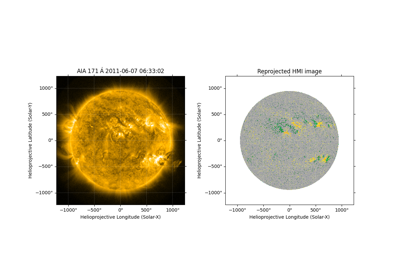Aligning AIA and HMI Data with Reproject

Aligning AIA and HMI Data with Reproject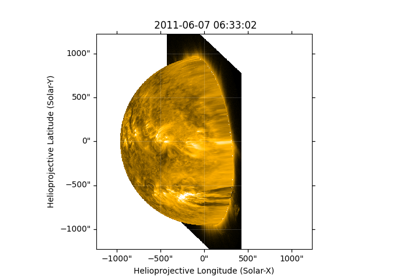Reprojecting Using a Spherical Screen

Reprojecting Using a Spherical ScreenBlending maps using mplcairo

Blending maps using mplcairo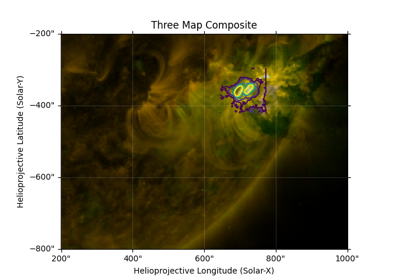Creating a Composite Plot with Three Maps

Creating a Composite Plot with Three Maps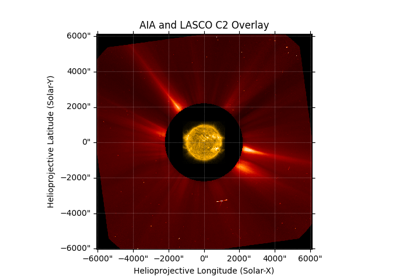Overlay an AIA image on a LASCO C2 coronagraph

Overlay an AIA image on a LASCO C2 coronagraph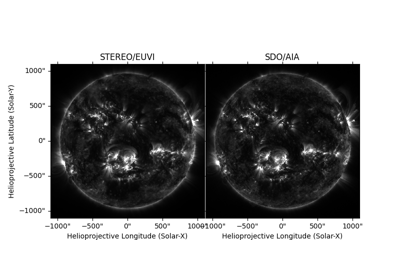Visualizing 3D stereoscopic images

Visualizing 3D stereoscopic images
>>> import astropy.units as u
>>> from sunpy.coordinates import Helioprojective
>>> h = Helioprojective(range(7)*u.arcsec*319, *7*u.arcsec,
...                     observer='earth', obstime='2020-04-08')
>>> print(h.make_3d())
<Helioprojective Coordinate (obstime=2020-04-08T00:00:00.000, rsun=695700.0 km, observer=<HeliographicStonyhurst Coordinate for 'earth'>): (Tx, Ty, distance) in (arcsec, arcsec, AU)
[(   0., 0., 0.99660825), ( 319., 0., 0.99687244),
( 638., 0., 0.99778472), ( 957., 0., 1.00103285),
(1276., 0.,        nan), (1595., 0.,        nan),
(1914., 0.,        nan)]>

>>> with Helioprojective.assume_spherical_screen(h.observer):
...     print(h.make_3d())
<Helioprojective Coordinate (obstime=2020-04-08T00:00:00.000, rsun=695700.0 km, observer=<HeliographicStonyhurst Coordinate for 'earth'>): (Tx, Ty, distance) in (arcsec, arcsec, AU)
[(   0., 0., 1.00125872), ( 319., 0., 1.00125872),
( 638., 0., 1.00125872), ( 957., 0., 1.00125872),
(1276., 0., 1.00125872), (1595., 0., 1.00125872),
(1914., 0., 1.00125872)]>

>>> with Helioprojective.assume_spherical_screen(h.observer, only_off_disk=True):
...     print(h.make_3d())
<Helioprojective Coordinate (obstime=2020-04-08T00:00:00.000, rsun=695700.0 km, observer=<HeliographicStonyhurst Coordinate for 'earth'>): (Tx, Ty, distance) in (arcsec, arcsec, AU)
[(   0., 0., 0.99660825), ( 319., 0., 0.99687244),
( 638., 0., 0.99778472), ( 957., 0., 1.00103285),
(1276., 0., 1.00125872), (1595., 0., 1.00125872),
(1914., 0., 1.00125872)]>

is_visible(*, tolerance: Unit("m") = <Quantity 1. m>)[source]#

Returns whether the coordinate is on the visible side of the Sun.

A coordinate is visible if it can been seen from the observer (the observer frame attribute) assuming that the Sun is an opaque sphere with a fixed radius (the rsun frame attribute). The visible side of the Sun is always smaller than a full hemisphere.

Parameters:

tolerance (Quantity) – The depth below the surface of the Sun that should be treated as transparent.

Notes

If the coordinate is 2D, it is automatically deemed visible. A 2D coordinate describes a look direction from the observer, who would simply see whatever is in “front”, and thus cannot correspond to a point hidden from the observer.

The tolerance parameter accommodates situations where the limitations of numerical precision would falsely conclude that a coordinate is not visible. For example, a coordinate that is expressly created to be on the solar surface may be calculated to be slightly below the surface, and hence not visible if there is no tolerance. However, a consequence of the tolerance parameter is that a coordinate that is formally on the far side of the Sun but is extremely close to the solar limb can be evaluated as visible. With the default tolerance value of 1 meter, a coordinate on the surface of the Sun can be up to 11 arcseconds of heliographic longitude past the solar limb and still be evaluated as visible.

Examples

>>> import numpy as np
>>> import astropy.units as u
>>> from astropy.coordinates import SkyCoord
>>> from sunpy.coordinates import HeliographicStonyhurst, Helioprojective

>>> hpc_frame = Helioprojective(observer='earth', obstime='2023-08-03')

>>> in_front = SkyCoord(0*u.arcsec, 0*u.arcsec, 0.5*u.AU, frame=hpc_frame)
>>> print(in_front.is_visible())
True

>>> behind = SkyCoord(0*u.arcsec, 0*u.arcsec, 1.5*u.AU, frame=hpc_frame)
>>> print(behind.is_visible())
False

>>> hgs_array = SkyCoord(np.arange(-180, 180, 60)*u.deg, *6*u.deg,
...                      frame='heliographic_stonyhurst', obstime='2023-08-03')
>>> print(hgs_array)
<SkyCoord (HeliographicStonyhurst: obstime=2023-08-03T00:00:00.000, rsun=695700.0 km): (lon, lat) in deg
[(-180., 0.), (-120., 0.), ( -60., 0.), (   0., 0.), (  60., 0.),
( 120., 0.)]>
>>> print(hgs_array.transform_to(hpc_frame).is_visible())
[False False  True  True  True False]

make_3d()[source]#

This method calculates the third coordinate of the Helioprojective frame. It assumes that the coordinate point is on the surface of the Sun.

If a point in the frame is off limb then NaN will be returned.

Returns:

new_frame (Helioprojective) – A new frame instance with all the attributes of the original but now with a third coordinate.